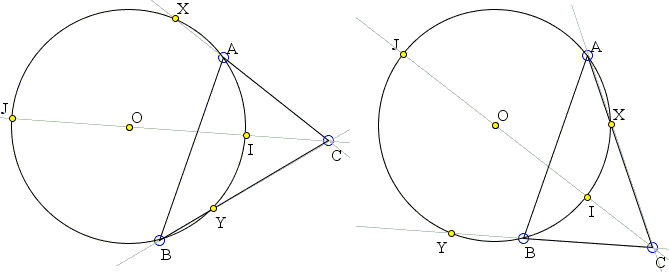# Circle through the Incenter

The applet below illustrates problem 7 from the 2009 Australian Mathematical Olympiad.

Let $I$ be the incenter of $\Delta ABC$ in which $AC \ne BC.$ Let $\Gamma$ be the circle passing through $A,$ $I$ and $B.$ Suppose $\Gamma$ intersects the line $AC$ at $A$ and $X$ and intersects the line $BC$ at $B$ and $Y.$ Show that $AX = BY.$

The condition $AC \ne BC$ is obviously a red herring as, in this case, $A = X$ and $B = Y.$

SolutionLet $I$ be the incenter of $\Delta ABC$ in which $AC \ne BC.$ Let $\Gamma$ be the circle passing through $A,$ $I$ and $B.$ Suppose $\Gamma$ intersects the line $AC$ at $A$ and $X$ and intersects the line $BC$ at $B$ and $Y.$ Show that $AX = BY.$

Two configurations are possible: one with $Y$ inside $BC$ and $X$ outside $AC$ and the other with $Y$ outside $BC$ and $X$ inside $AC.$Without loss of generality, we shall consider just one of them. This is illustrated below:In the diagram, $J$ is the other intersection of the bisector $CI$ with the circle and, for simplicity, the arcs are enumerated, so that, in the following $I$ shall refer to the arcs by those numbers: $IY = 1,$ $AI = 2,$ $AX = 3,$ $JX = 4,$ $BJ = 5,$ $BY = 6.$ Angles of $\Delta ABC$ are $2\alpha,$ $2\beta,$ $2\gamma\;$ and $\angle AIB = \delta .$

The problem is solved through angle calculations, commonly referred to as "angle chasing". The fact that $I$ is the incenter of $\Delta ABC$ provides sufficient reasons and grounds to insure the satisfactory outcome.

First of all, in $\Delta ABC,$ $2\alpha + 2\beta + 2\gamma = 180^{\circ}$ whereas, in $\Delta AIB,$ $\alpha + \beta + \delta = 180^{\circ}.$ This gives $\delta = 90^{\circ} + \gamma .$ Since $AIB$ is an inscribed angle we get one arc relation:

(1)

$3 + 4 + 5 = 180^{\circ} + 2\gamma .$

Next, $\angle ACJ = \angle BCJ = \gamma .$ From the properties of the angles between two secants,

(2)

$4 - 2 = 5 - 1 = 2\gamma .$

Since $BI$ is the angle bisector, $\angle ABI = \angle CBI = \beta ,$ from which

(3)

$1 = 2 = 2\beta .$

Together with (2) this implies

(4)

$4 = 5 = 2\gamma + 2\beta .$

Further, $\angle BAI = \alpha$ and is subtended by the arc $BI$ so that

(5)

$1 + 6 = 2\alpha .$

Combining this with (3) gives

(6)

$6 = 2\alpha - 2\beta .$

On the other hand, from (1) and (4),

(7)

$3 = 180^{\circ} - 2\gamma - 4\beta .$

Together, (6) and (7) show that $3 = 6,$ as required. (Indeed, the identity checks out: $2\alpha - 2\beta = 180^{\circ} - 2\gamma - 4\beta$ is equivalent to $2\alpha + 2\beta + 2\gamma = 180^{\circ}.)$

(A different proof has been posted by Vo Duc Dien at the wiki part of the site.)

One engaging interpretation of this result is that

A circle through two vertices of a triangle and its incenter is always centered on the angle bisector of the remaining vertex and is therefore symmetric in that angle bisector.

Another solution makes a better use of this observation.

There are five solutions in all:### What Is Red Herring